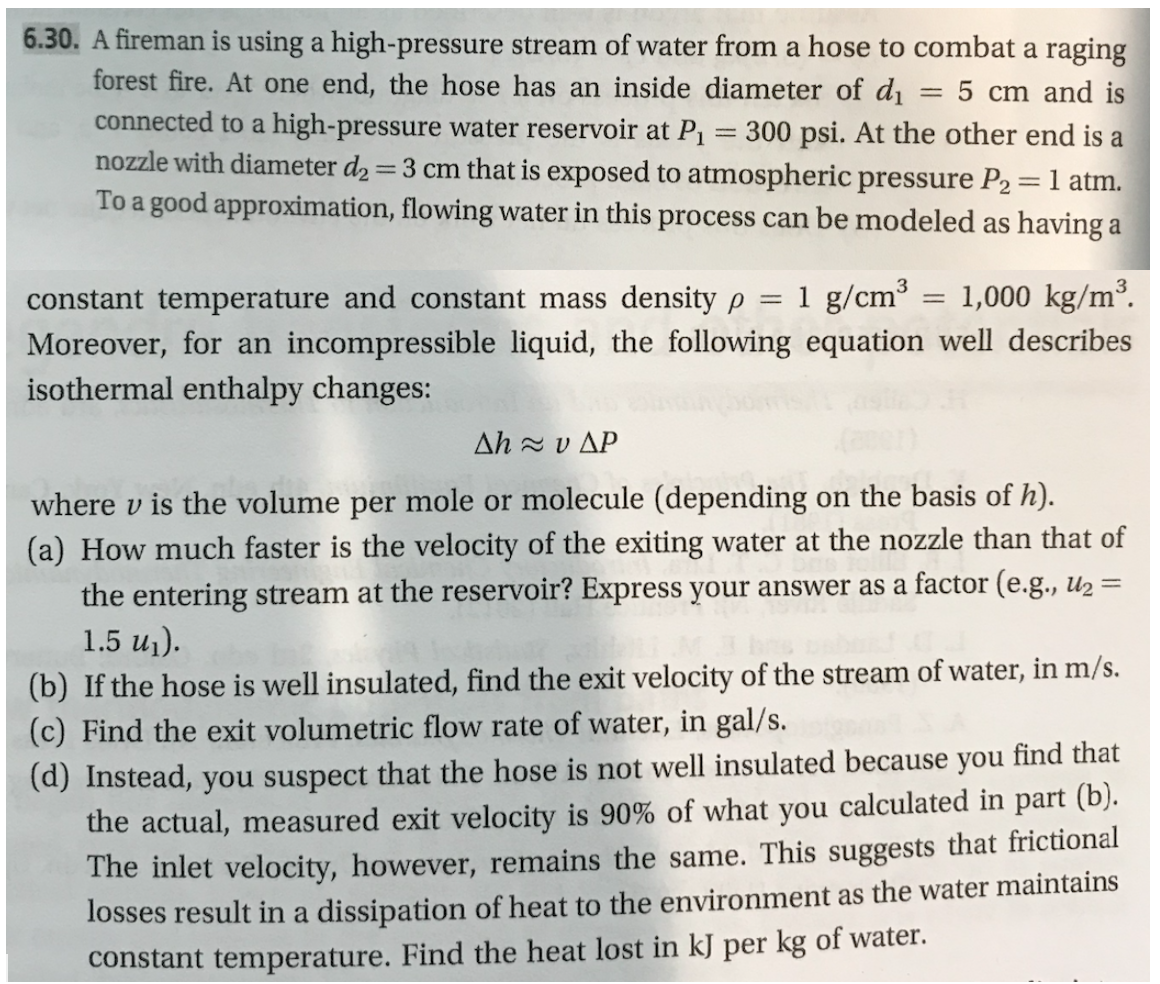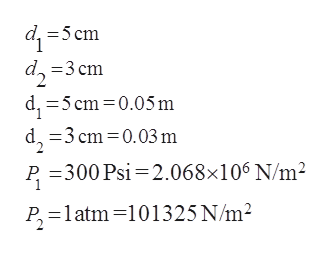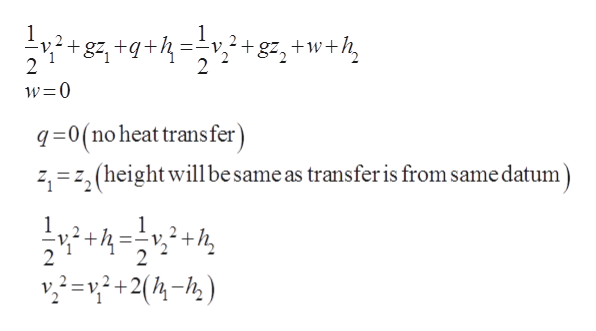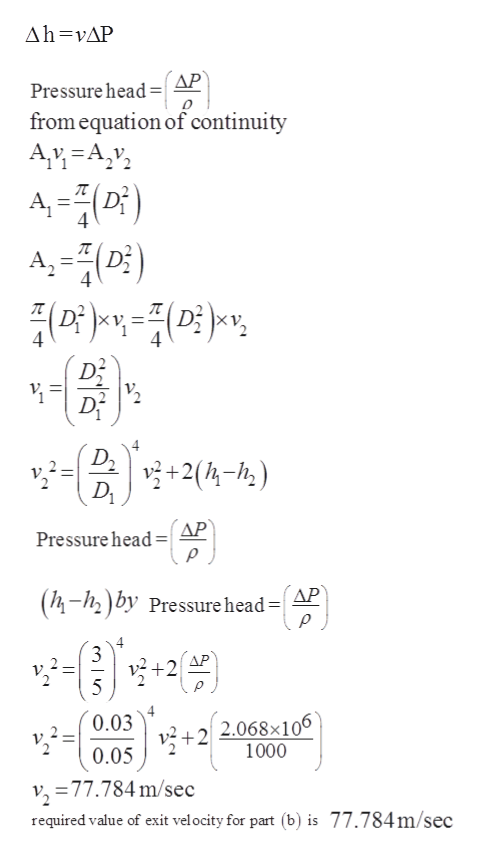6.30. A fireman is using a high-pressure stream of water from a hose to combat a ragingforest fire. At one end, the hose has an inside diameter of dconnected to a high-pressure water reservoir at P1 300 psi. At the other end is anozzle with diameter d2 3 cm that is exposed to atmospheric pressure P2= 1 atmTo a good approximation, flowing water in this process can be modeled as having a5 cm and is3constant temperature and constant mass density p = 1 g/cm = 1,000 kg/m2.incompressible liquid, the following equation well describesMoreover, for anisothermal enthalpy changes:Δh xυΔΡwhere v is the volume per mole or molecule (depending(a) How much faster is the velocity of the exitingon the basis of h)water at the nozzle than that offactor (e.g., u2=the entering stream at the reservoir? Express your answer as a1.5 u1).(b) If the hose is well insulated, find the exit velocity of the stream of water, in m/s.(c) Find the exit volumetric flow rate of water, in gal/s.(d) Instead, you suspect that the hose is not well insulated because you find thatthe actual, measured exit velocity is 90% of what you calculated in part (b).The inlet velocity, however, remains the same. This suggests that frictionallosses result in a dissipation of heat to the environment as the water maintainsconstant temperature. Find the heat lost in kJ per kg of water

Question

This problem is (6.30) from a book  "Thermodynamics and Statistical Mechanics An Integrated Approach by M. Scott Shell"help_outlineImage Transcriptionclose6.30. A fireman is using a high-pressure stream of water from a hose to combat a raging forest fire. At one end, the hose has an inside diameter of d connected to a high-pressure water reservoir at P1 300 psi. At the other end is a nozzle with diameter d2 3 cm that is exposed to atmospheric pressure P2= 1 atm To a good approximation, flowing water in this process can be modeled as having a 5 cm and is 3 constant temperature and constant mass density p = 1 g/cm = 1,000 kg/m2. incompressible liquid, the following equation well describes Moreover, for an isothermal enthalpy changes: Δh xυΔΡ where v is the volume per mole or molecule (depending (a) How much faster is the velocity of the exiting on the basis of h) water at the nozzle than that of factor (e.g., u2= the entering stream at the reservoir? Express your answer as a 1.5 u1). (b) If the hose is well insulated, find the exit velocity of the stream of water, in m/s. (c) Find the exit volumetric flow rate of water, in gal/s. (d) Instead, you suspect that the hose is not well insulated because you find that the actual, measured exit velocity is 90% of what you calculated in part (b). The inlet velocity, however, remains the same. This suggests that frictional losses result in a dissipation of heat to the environment as the water maintains constant temperature. Find the heat lost in kJ per kg of water fullscreen
Step 1

Hose is used for conveying water with the value of inside and outside diameter along with the given datahelp_outlineImage Transcriptionclosed5cm d, 3 cm d5 cm 0.05 m d 3cm 0.03 m P 300 Psi 2.068x106N/m2 P 1atm-101325 N/m2 fullscreen
Step 2

First for part (b) the applying energy balance

Energy balanced based on the amount of kinetic energy, potential energy and the amount of heat generated

Taking the datum on same level

On further solving we havehelp_outlineImage Transcriptionclose1 2 -V -v -+ 2 + 1 2 w 0 q0(no heat trans fer) 2 2 (heightwilbe same as transfer is from same datum 2 2 222(2) fullscreen
Step 3

As per given information

Pressure head is defined as the ratio of pressure difference to density

using the con...help_outlineImage TranscriptioncloseAh vAP AP Pressure head= from equation of continuity Av A,v A-(D) A,() 4 4 4 4 D+2(4-h) Pressure head AP (4-12) by Pressure head=| AP 4 3 AP 5 0.03 2 2.068x106 1000 +2 0.05 v, 77.784m/sec required value of exit velocity for part (b) is 77.784m/sec fullscreen

Want to see the full answer?

See Solution

Want to see this answer and more?

Our solutions are written by experts, many with advanced degrees, and available 24/7

See Solution
Tagged in

Chemical Engineering